## SQL

 Question 1

A relational database contains two tables Student and Performance as shown below:The primary key of the Student table is Roll_no. For the Performance table, the columns Roll_no. and Subject_code together from the primary key. Consider the SQL query given below:

```SELECT S.Student_name, sum (P.Marks)
FROM Student S, Performance P
WHERE P.Marks > 84
GROUP BY S.Student_name;```

The number of rows returned by the above SQL query is _____.

 A 0 B 9 C 7 D 5
Database-Management-System       SQL       GATE 2019       Video-Explanation
Question 1 Explanation:
(Executed under Oracle Express Edition)
SQL> SELECT S.Student_name,sum(P.Marks)
2 FROM Student S,Performance P
3 WHERE P.Marks>84
4 GROUP BY S.Student_name;Question 2

Consider the following two tables and four queries in SQL.

Book (isbn, bname), Stock (isbn, copies)
```Query 1:    SELECT B.isbn, S.copies
FROM Book B INNER JOIN Stock S
ON B.isbn = S.isbn;

Query 2:    SELECT B.isbn, S.copies
FROM Book B LEFT OUTER JOIN Stock S
ON B.isbn = S.isbn;

Query 3:    SELECT B.isbn, S.copies
FROM Book B RIGHT OUTER JOIN Stock S
ON B.isbn = S.isbn;

Query 4:    SELECT B.isbn, S.copies
FROM Book B FULL OUTER JOIN Stock S
ON B.isbn = S.isbn;
```

Which one of the queries above is certain to have an output that is a superset of the outputs of the other three queries?

 A Query 1 B Query 2 C Query 3 D Query 4
Database-Management-System       SQL       GATE 2018       Video-Explanation
Question 2 Explanation:
Given two tables are,
Book (isbn, bname)
Stock (isbn, copies)
isbn is a primary key of Book and isbn is a foreign key of stock referring to Book table.
For example:Query 1:
INNER JOIN keyword selects records that have matching values in both tables (Book and Stock).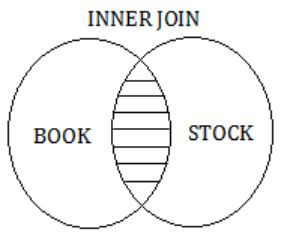So, the result of Query 1 is,Query 2:
The LEFT OUTER JOIN keyword returns all records from the left table (Book) and the matched records from the right table (Stock).
The result is NULL from the right side, if there is no match.So, the result of Query 2 is,Query 3:
The RIGHT OUTER JOIN keyword returns all records from the right table (Stock), and the matched records from the left table(BOOK).
The result is NULL from the left side, when there is no match.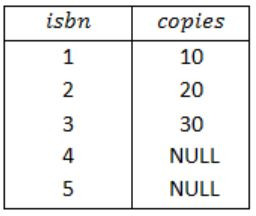Query 4:
The FULL OUTER JOIN keyword return all records when there is a match in either left (Book) or right (Stock) table records.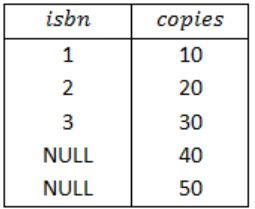So, the result of Query 4 is,Therefore, from the result of above four queries, a superset of the outputs of the Query 1, Query 2 and Query 3 is Query 4.
Note:
If we take isbn as a primary key in both the tables Book and Stock and foreign key, in one of the tables then also will get option (D) as the answer.
 Question 3

Consider a database that has the relation schema EMP (EmpId, EmpName, and DeptName). An instance of the schema EMP and a SQL query on it are given below.The output of executing the SQL query is ___________.

 A 2.6 B 2.7 C 2.8 D 2.9
Database-Management-System       SQL       GATE 2017 [Set-1]       Video-Explanation
Question 3 Explanation:
The given query is⇾ We start evaluating from the inner query.
The inner query forms DeptName wise groups and counts the DeptName wise EmpIds.
⇾ In inner query DeptName, Count(EmpId) is the alias name DeptName, Num.
So, the output of the inner query is,The outer query will find the
Avg(Num) = (4+3+3+2+1)/5 = 2.6
 Question 4

Consider the following database table named top_scorer.```SELECT ta.player FROM top_scorer AS ta
WHERE ta.goals > ALL (SELECT tb.goals
FROM top_scorer AS tb
WHERE tb.country = 'Spain')
AND ta.goals > ANY (SELECT tc.goals
FROM top_scorer AS tc
WHERE tc.country = 'Germany')```

The number of tuples returned by the above SQL query is _____.

 A 7 B 8 C 9 D 10
Database-Management-System       SQL       GATE 2017 [Set-2]       Video-Explanation
Question 4 Explanation:In the given database table top_scorer no players are there from ‘Spain’.
So, the query (1) results 0 and ALL (empty) is always TRUE.
The query (2) selects the goals of the players those who are belongs to ‘Germany’.
So, it results in ANY (16, 14, 11, 10).
So, the outer most query results the player names from top_scorer, who have more goals.
Since, the minimum goal by the ‘Germany’ player is 10, it returns the following 7 rows.Question 5

Consider the following database table named water_schemes :The number of tuples returned by the following SQL query is _________.

```with total(name, capacity) as
select district_name, sum(capacity)
from water_schemes
group by district_name
with total_avg(capacity) as
select avg(capacity)
from total
select name
from total, total_avg
where total.capacity ≥ total_avg.capacity
```
 A 2 B 3 C 4 D 5
Database-Management-System       SQL       GATE 2016 [Set-2]       Video-Explanation
Question 5 Explanation:
• The SQL WITH clause allows you to give a sub-query block a name (a process also called sub-query refactoring), which can be referenced in several places within the main SQL query.
The name assigned to the sub-query is treated as though it was an inline view or table.
• First group by district name is performed and total capacities are obtained as following:• Then average capacity is computed,
Average Capacity = (20 + 40 + 30 + 10)/4
= 100/4
= 25
• Finally, 3rd query will be executed and it's tuples will be considered as output, where name of district and its total capacity should be more than or equal to 25.
• Then average capacity is computed,
Average Capacity = (20 + 40 + 30 + 10)/4
= 100/4
= 25
• Finally, 3rd query will be executed and it's tuples will be considered as output, where name of district and its total capacity should be more than or equal to 25.Question 6

SELECT operation in SQL is equivalent to

 A the selection operation in relational algebra B the selection operation in relational algebra, except that SELECT in SQL retains duplicates C the projection operation in relational algebra D the projection operation in relational algebra, except that SELECT in SQL retains duplicates
Database-Management-System       SQL       GATE 2015 [Set-1]
Question 6 Explanation:
SELECT operation in SQL perform vertical partitioning which is performed by projection operation in relational calculus but SQL is multi sets; hence (D).
 Question 7

Consider the following relations:Consider the following SQL query.

```SELECT S. Student_Name, sum(P.Marks)
FROM Student S, Performance P
WHERE S.Roll_No = P.Roll_No
GROUP BY S.Student_Name```

The number of rows that will be returned by the SQL query is _________.

 A 2 B 3 C 4 D 5
Database-Management-System       SQL       GATE 2015 [Set-1]
Question 7 Explanation:
Output table isQuestion 8

Consider the following relation

`  Cinema (theater, address, capacity)`

Which of the following options will be needed at the end of the SQL query

```SELECT P1. address
FROM Cinema P1```

Such that it always finds the addresses of theaters with maximum capacity?

 A WHERE P1.capacity >= All (select P2.capacity from Cinema P2) B WHERE P1.capacity >= Any (select P2.capacity from Cinema P2) C WHERE P1.capacity > All (select max(P2.capacity) from Cinema P2) D WHERE P1.capacity > Any (select max(P2.capacity) from Cinema P2)
Database-Management-System       SQL       GATE 2015 [Set-3]
Question 8 Explanation:
Inner query collects capacities of all the theaters and in outer query we are filtering the tuples with the condition “capacity >= All”.
So the theaters which are having maximum capacity will satisfy the condition.
 Question 9

Given the following statements:

```    S1: A foreign key declaration can always
be replaced by an equivalent check
assertion in SQL.
S2: Given the table R(a,b,c) where a and
b together form the primary key, the
following is a valid table definition.
CREATE TABLE S (
a INTEGER,
d INTEGER,
e INTEGER,
PRIMARY KEY (d),
FOREIGN KEY (a) references R)```

Which one of the following statements is CORRECT?

 A S1 is TRUE and S2 is FALSE. B Both S1 and S2 are TRUE. C S1 is FALSE and S2 is TRUE. D Both S1 and S2 are FALSE.
Database-Management-System       SQL       GATE 2014 [Set-1]
Question 9 Explanation:
S1: False
Using a check constraint, we can have the same effect as foreign key while adding elements to the child table. But while deleting elements from the parent table the referential integrity constraint is no longer valid. So, a check constraint cannot replace a foreign key.
S2: False:
Foreign key in one table should be defined as a primary key in other table. In above table definition, table S has a foreign key that refers to field ‘a’ of R. The field ‘a’ in table S is part of the primary key and part of the key cannot be declared as a foreign key.
 Question 10

Given the following schema:

```     employees(emp-id, first-name, last-name, hire-date, dept-id, salary)
departments(dept-id, dept-name, manager-id, location-id)```

You want to display the last names and hire dates of all latest hires in their respective departments in the location ID 1700. You issue the following query:

```SQL> SELECT last-name, hire-date
FROM employees
WHERE (dept-id, hire-date) IN ( SELECT dept-id, MAX(hire-date)
FROM employees JOIN departments USING(dept-id)
WHERE location-id = 1700
GROUP BY dept-id); ```

What is the outcome?

 A It executes but does not give the correct result. B It executes and gives the correct result. C It generates an error because of pairwise comparison. D It generates an error because the GROUP BY clause cannot be used with table joins in a subquery.
Database-Management-System       SQL       GATE 2014 [Set-1]
Question 10 Explanation:
The given SQL query will display the last names and hire-dates of all latest hires in their respective departments in the location ID 1700. So, correct option is (B).
 Question 11

SQL allows tuples in relations, and correspondingly defines the multiplicity of tuples in the result of joins. Which one of the following queries always gives the same answer as the nested query shown below:

`    select * from R where a in (select S.a from S)`
 A select R.* from R,S where R.a=S.a B select distinct R.* from R,S where R.a=S.a C select R.* from R,(select distinct a from S) as S1 where R.a=S1.a D select R.* from R,S where R.a=S.a and is unique R
Database-Management-System       SQL       GATE 2014 [Set-2]
Question 11 Explanation:
Multiplicity of duplicate tuples will be distributed when there is a match between R.a and S.a; and for that match, S.a's value is repeated in each cases except the third case. So, the output of query given in the question matches with the output of (C).
 Question 12

Consider the following relational schema:

```  employee(empId, empName, empDept)
customer(custId, custName, salesRepId, rating)```

salesRepId is a foreign key referring to empId of the employee relation. Assume that each employee makes a sale to at least one customer. What does the following query return?

```SELECT empName
FROM employee E
WHERE NOT EXISTS (SELECT custId
FROM customer C
WHERE C.salesRepId = E.empId
AND C.rating <> `GOOD`); ```
 A Names of all the employees with at least one of their customers having a ‘GOOD’ rating. B Names of all the employees with at most one of their customers having a ‘GOOD’ rating. C Names of all the employees with none of their customers having a ‘GOOD’ rating. D Names of all the employees with all their customers having a ‘GOOD’ rating.
Database-Management-System       SQL       GATE 2014 [Set-3]
Question 12 Explanation:The inner query i.e., ② represents all customers having other than ‘GOOD’ while the entire query represents name of all employees with all their customers having a ‘good rating’.
 Question 13

Which of the  following statements are TRUE about an SQL query?

P:An SQL query can contain a HAVING clause even if it does not have a GROUP BY clause.
Q:An SQL query can contain a HAVING clause even if it has a GROUP BY clause.
R: All attributes used in the GROUP BY clause must appear in the SELECT clause.
S: Not all attributes used in the GROUP BY clause need to appear in the SELECT clause
 A P and R B P and S C Q and R D Q and S
Database-Management-System       SQL       GATE 2012
Question 13 Explanation:
The SQL GROUP BY clause is used in collaboration with the SELECT statement to arrange identical data into groups. This GROUP BY clause follows the WHERE clause in a SELECT statement and precedes the ORDER BY clause. The attributes used in GROUP BY clause must present in SELECT statement.
The HAVING Clause enables you to specify conditions that filter which group results appear in the results. The WHERE clause places conditions on the selected columns, whereas the HAVING clause places conditions on groups created by the GROUP BY clause. So, we cannot use HAVING clause without GROUP BY clause.
 Question 14

Consider the following relations A, B, C.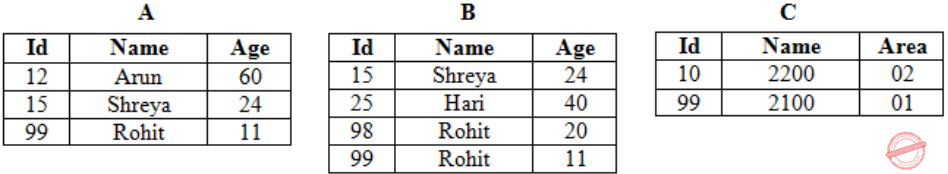How many tuples does the result of the following SQL query contain?

```SELECT A.id
FROM A
WHERE A.age > ALL (SELECT B.age
FROM B
WHERE B. name = "arun")```
 A 4 B 3 C 0 D 1
Database-Management-System       SQL       GATE 2012
Question 14 Explanation: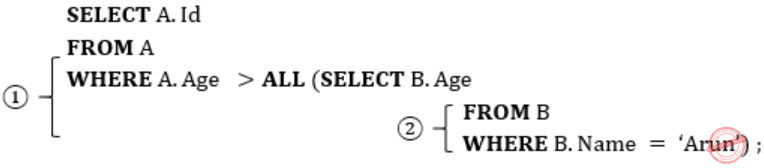First query (2) will be executed and 0 (no) rows will be selected because in relation B there is no Name ‘Arun’.
The outer query (1) results the follow and that will be the result of entire query now. (Because inner query returns 0 rows).Question 15

Database table by name Loan_Records is given below.

```Borrower    Bank_Manager   Loan_Amount
Ramesh      Sunderajan     10000.00
Suresh      Ramgopal       5000.00
Mahesh      Sunderajan     7000.00```
What is the output of the following SQL query?
```SELECT Count(*)
FROM  (
(SELECT Borrower, Bank_Manager FROM Loan_Records) AS S
NATURAL JOIN
(SELECT Bank_Manager, Loan_Amount FROM Loan_Records) AS T
);```
 A 3 B 9 C 5 D 6
Database-Management-System       SQL       GATE 2011
Question 15 Explanation:Question 16

Consider a database table T containing two columns X and Y each of type integer. After the creation of the table, one record (X=1, Y=1) is inserted in the table. Let MX and My denote the respective maximum values of X and Y among all records in the table at any point in time. Using MX and MY, new records are inserted in the table 128 times with X and Y values being MX+1, 2*MY+1 respectively. It may be noted that each time after the insertion, values of MX and MY change. What will be the output of the following SQL query after the steps mentioned above are carried out?

`SELECT Y FROM T WHERE X=7;`
 A 127 B 255 C 129 D 257
Database-Management-System       SQL       GATE 2011
Question 16 Explanation:Question 17

A relational schema for a train reservation database is given below. Passenger (pid, pname, age) Reservation (pid, class, tid)

```Table: Passenger
pid   pname   age
-----------------
0    Sachin   65
1    Rahul    66
2    Sourav   67
3    Anil     69

Table : Reservation
pid  class  tid
---------------
0    AC   8200
1    AC   8201
2    SC   8201
5    AC   8203
1    SC   8204
3    AC   8202```

What pids are returned by the following SQL query for the above instance of the tables?

```SELECT pid
FROM Reservation ,
WHERE class ‘AC’ AND
EXISTS (SELECT *
FROM Passenger
WHERE age > 65 AND
Passenger. pid = Reservation.pid)```
 A 1, 0 B 1, 2 C 1, 3 D 1, 5
Database-Management-System       SQL       GATE 2010
Question 17 Explanation:
Passenger: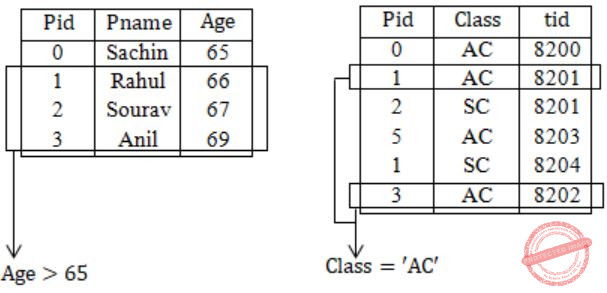― 1, 3 Pids are returned
 Question 18

Consider the following relational schema:

```
Suppliers(sid:integer, sname:string, city:string, street:string)
Parts(pid:integer, pname:string, color:string)
Catalog(sid:integer, pid:integer, cost:real)```

Consider the following relational query on the above database:

```        SELECT S.sname
FROM Suppliers S
WHERE S.sid NOT IN (SELECT C.sid
FROM Catalog C
WHERE C.pid NOT IN (SELECT P.pid
FROM Parts P
WHERE P.color <> 'blue'))```

Assume that relations corresponding to the above schema are not empty. Which one of the following is the correct interpretation of the above query?

 A Find the names of all suppliers who have supplied a non-blue part. B Find the names of all suppliers who have not supplied a non-blue part. C Find the names of all suppliers who have supplied only blue parts. D Find the names of all suppliers who have not supplied only blue parts.
Database-Management-System       SQL       GATE 2009
Question 18 Explanation:If we execute the given query the output will be S3 and S4 i.e., names of all suppliers who didn’t supply blue parts which is option (A).
Option (D) says names of suppliers who didn’t supply only blue parts that means, supplier should supply all other parts for sure and shouldn’t supply blue part.
 Question 19

Consider the table employee(empId, name, department, salary) and the two queries Q1,Q2 below. Assuming that department 5 has more than one employee, and we want to find the employees who get higher salary than anyone in the department 5, which one of the statements is TRUE for any arbitrary employee table?

```Q1 : Select e.empId
From employee e
Where not exists
(Select * From employee s where s.department = “5” and s.salary >= e.salary)
Q2 : Select e.empId
From employee e
Where e.salary > Any
(Select distinct salary From employee s Where s.department = “5”)```
 A Q1 is the correct query B Q2 is the correct query C Both Q1 and Q2 produce the same answer D Neither Q1 nor Q2 is the correct query
Database-Management-System       SQL       GATE 2007
Question 19 Explanation:
The required output is find the employees who get higher salary than anyone in the department “5”.
Query 1: Results the empId's which have higher salary than anyone in the department 5.
Query 2: Results the empId's which have higher salary than atleast one employee of department 5.
 Question 20

Consider the relation account (customer, balance) where customer is a primary key and there are no null values. We would like to rank customers according to decreasing balance. The customer with the largest balance gets rank 1. ties are not broke but ranks are skipped: if exactly two customers have the largest balance they each get rank 1 and rank 2 is not assigned.

```Query1:
select A.customer, count(B.customer)
from account A, account B
where A.balance <=B.balance
group by A.customer

Query2:
select A.customer, 1+count(B.customer)
from account A, account B
where A.balance < B.balance
group by A.customer```

Consider these statements about Query1 and Query2.

```1. Query1 will produce the same row set as Query2 for
some but not all databases.
2. Both Query1 and Query2 are correct implementation
of the specification
3. Query1 is a correct implementation of the specification
but Query2 is not
4. Neither Query1 nor Query2 is a correct implementation
of the specification
5. Assigning rank with a pure relational query takes
less time than scanning in decreasing balance order
assigning ranks using ODBC.```

Which two of the above statements are correct?

 A 2 and 5 B 1 and 3 C 1 and 4 D 3 and 5
Database-Management-System       SQL       GATE 2006
Question 20 Explanation:
Query 1 & 2 gives the same output for all not all data based its true because the salaries may be distinct variables. Statement 1 is true.
The customer with largest balance gets rank 1. Ties are broken with ranks are skipped.
So, both queries may doesn’t give same output. Statement 4 is correct.
 Question 21

Consider the relation "enrolled(student, course)" in which (student, course) is the primary key, and the relation "paid(student, amount)" where student is the primary key. Assume no null values and no foreign keys or integrity constraints. Given the following four queries:

```Query1: select student from enrolled where student in (select student from paid)
Query2: select student from paid where student in (select student from enrolled)
Query3: select E.student from enrolled E, paid P where E.student = P.student
Query4: select student from paid where exists
(select * from enrolled where enrolled.student = paid.student)```

Which one of the following statements is correct?

 A All queries return identical row sets for any database. B Query2 and Query4 return identical row sets for all databases but there exist databases for which Query1 and Query2 return different row sets. C There exist databases for which Query3 returns strictly fewer rows than Query2. D There exist databases for which Query4 will encounter an integrity violation at runtime.
Database-Management-System       SQL       GATE 2006
Question 21 Explanation:
Consider Table examples as:Query1 : Output
abcd
abcd
PQRS
Query 2 : Output
abcd
PQRS
Query 3 : Output
abcd
PQRS
Query 4 : Output
abcd
PQRS
Query 2 & Query 4 gives same results but Query 1 & Query 3 gives different results.
 Question 22

Consider the relation enrolled(student, course) in which (student, course) is the primary key, and the relation paid(student, amount), where student is the primary key. Assume no null values and no foreign keys or integrity constraints. Assume that amounts 6000, 7000, 8000, 9000 and 10000 were each paid by 20% of the students. Consider these query plans (Plan 1 on left, Plan 2 on right) to "list all courses taken by students who have paid more than x".A disk seek takes 4ms, disk data transfer bandwidth is 300 MB/s and checking a tuple to see if amount is greater than x takes 10 micro-seconds. Which of the following statements is correct?

 A Plan 1 and Plan 2 will not output identical row sets for all databases. B A course may be listed more than once in the output of Plan 1 for some databases. C For x = 5000, Plan 1 executes faster than Plan 2 for all databases. D For x = 9000, Plan I executes slower than Plan 2 for all databases.
Database-Management-System       SQL       GATE 2006
Question 22 Explanation:
Both plans are require the tables such as courses and enrolled to access the disks takes same time for both plans.
While analyze the plan1 and plan2 does lesser number of comparisons compared to plan1.
i) The join table consists of two tables will have more rows. So comparisons are needed to find amount greater than x.
ii) Join operation consists of more number of comparisons as the second table will have more rows in plan2 compared to plan1.
 Question 23
The relation book (title, price) contains the titles and prices of different books. Assuming that no two books have the same price, what does the following SQL query list?
```     select title
from book as B
where (select count(*)
from book as T
where T.price > B.price) < 5 ```
 A Titles of the four most expensive books B Title of the fifth most inexpensive book C Title of the fifth most expensive book D Titles of the five most expensive books
Database-Management-System       SQL       GATE 2005
Question 23 Explanation:
Which results titles of the five most expensive books.
The where clause of outer query will be true for 5 most expensive books.
 Question 24

The employee information in a company is stored in the relation

```Employee (name, sex, salary, deptName)
Consider the following SQL query
Select deptName
From Employee
Where sex = 'M'
Group by deptName
Having avg (salary) >
(select avg (salary) from Employee)```

It returns the names of the department in which

 A the average salary is more than the average salary in the company B the average salary of male employees is more than the average salary of all male employees in the company C the average salary of male employees is more than the average salary of employees in the same department D the average salary of male employees is more than the average salary in the company
Database-Management-System       SQL       GATE 2004
Question 24 Explanation:
Group by (avg(salary) > (select avg (salary) from employee))
This results the employees who having the salary more than the average salary.
Sex = M
Selects the Male employees whose salary is more than the average salary in the company.
 Question 25

Consider the following SQL query

```   select distinct al, a2,........., an
from rl, r2,........, rm
where P ```

For an arbitrary predicate P, this query is equivalent to which of the following relational algebra expressions?

 ABCDDatabase-Management-System       SQL       GATE 2003
Question 25 Explanation:
If we want to get distinct elements then we need to perform cross product in between the relations r1, r2, .... rm.
 Question 26

Consider the set of relations shown below and the SQL query that follows.

```  Students: (Roll_number, Name, Date_of_birth)
Courses: (Course number, Course_name, Instructor)
select distinct Name
and Courses.Instructor = Korth

Which of the following sets is computed by the above query?

 A Names of students who have got an A grade in all courses taught by Korth B Names of students who have got an A grade in all courses C Names of students who have got an A grade in at least one of the courses taught by Korth D in none of the above
Database-Management-System       SQL       GATE 2003
Question 26 Explanation:
The query results a names of students who got an A grade in at least one of the courses taught by korth.
 Question 27

Consider a relation geq which represents “greater than or equal to”, that is, (x,y) ∈ geq only if y >= x.

```create table geq
(  Ib integer not null
ub integer not null
primary key 1b
foreign key (ub) references geq on delete cascade ) ```

Which of the following is possible if a tuple (x,y) is deleted?

 A A tuple (z,w) with z > y is deleted B A tuple (z,w) with z > x is deleted C A tuple (z,w) with w < x is deleted D The deletion of (x,y) is prohibited
Database-Management-System       SQL       GATE 2001
 Question 28

Given relations r(w, x) and s(y, z), the result of

```    select distinct w, x
from r, s  ```

is guaranteed to be same as r, provided

 A r has no duplicates and s is non-empty B r and s have no duplicates C s has no duplicates and r is non-empty D r and s have the same number of tuples
Database-Management-System       SQL       GATE 2000
Question 28 Explanation:
r has no duplicate, if r can have duplicates it can be remove in the final state. s in non-empty if s is empty then r*s becomes empty.
 Question 29

In SQL, relations can contain null values, and comparisons with null values are treated as unknown. Suppose all comparisons with a null value are treated as false. Which of the following pairs is not equivalent?

 A x = 5 not AND (not (x = 5) B x = 5 AND x > 4 and x < 6, where x is an integer C x ≠ 5 AND not (x = 5) D None of the above
Database-Management-System       SQL       GATE 2000
Question 29 Explanation:
For all values less than five, x<5 is true and if x=5 then it is false.
 Question 30

Consider a bank database with only one relation
transaction (transno, acctno, date, amount)
The amount attribute value is positive for deposits and negative for withdrawals.

(a) Define an SQL view TP containing the information.
(acctno, T1.date, T2.amount)
for every pair of transactions T1, T2 such that T1 and T2 are transaction on the same account and the date of T2 is ≤ the date of T1.

(b) Using only the above view TP, write a query to find for each account the minimum balance it ever reached (not including the 0 balance when the account is created). Assume there is at most one transaction per day on each account, and each account has had atleast one transaction since it was created. To simply your query, break it up into 2 steps by defining an intermediate view V.

 A Theory Explanation is given below.
Database-Management-System       SQL       GATE 2000
 Question 31

Which of the following is/are correct?

 A An SQL query automatically eliminates duplicates B An SQL query will not work if there are no indexes on the relations C SQL permits attribute names to be repeated in the same relation D None of the above
Database-Management-System       SQL       GATE 1999
Question 31 Explanation:
→ SQL won't remove duplicates like relational algebra projection, we have to remove it explicitly by distinct.
→ If there are no indexes on the relation SQL, then also it works.
→ SQL does not permit 2 attributes to have same name in a relation.
 Question 32

Consider the set of relations

```EMP(Employee-no, Dept-no, Employee-name, Salary)
DEPT(Dept-no, Dept-name, Location) ```

Write an SQL query to:
(a) Find all employee names who work in departments located at "Calcutta" and whose salary is greater than Rs. 50,000.
(b) Calculate, for each department number, the number of employees with a salary greater than Rs. 100,000.

 A Theory Explanation.
Database-Management-System       SQL       GATE 1999
 Question 33

(a) Suppose we have a database consisting of the following three relations.
FREQUENTS(student, parlor) giving the parlors each student visits.
SERVES(parlor, ice-cream) indicating what kind of ice-creams each parlor serves.
LIKES(student, ice-cream) indicating what ice-creams each parlor serves.
(Assuming that each student likes at least one ice-cream and frequents at least one parlor)
Express the following in SQL:
Print the students that frequent at least one parlor that serves some ice-cream that they like.

(b) In a computer system where the 'best-fit' algorithm is used for allocating 'jobs' to 'memory partitions', the following situation was encountered: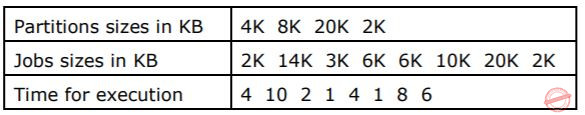When will the 20K job complete? Note - This question was subjective type.

 A Theory Explanation.
Database-Management-System       SQL       GATE 1998
 Question 34

Consider the following database relations containing the attributes

```Book_id
Subject_Category_of_book
Name_of_Author
Nationality_of_Author
with Book_id as the Primary Key. ```

(a) What is the highest normal form satisfied by this relation?

(b) Suppose the attributes Book_title and Author_address are added to the relation, and the primary key is changed to (Name_of_Author, Book_Title), what will be the highest normal form satisfied by the relation?

 A Theory Explanation.
Database-Management-System       SQL       GATE 1998
 Question 35

Consider the following relational database schemes:

```  COURSES(Cno, name)
PRE-REQ(Cno, pre_Cno)
COMPLETED(student_no, Cno) ```

COURSES give the number and the name of all the available courses.
PRE-REQ gives the information about which course are pre-requisites for a given course.
COMPLETED indicates what courses have been completed by students.

Express the following using relational algebra:
List all the courses for which a student with student_no 2310 has completed all the pre-requisites.

 A Theory Explanation.
Database-Management-System       SQL       GATE 1998
 Question 36

A library relational database system uses the following schema

```USERS (User#, UserName, HomeTown)
BOOKS (Book#, BookTitle, AuthorName)
ISSUED (Book#, User#, Date) ```

Explain in one English sentence, what each of the following relational algebra queries is designed to determine

```(a) σ User #=6 (11 User #, Book Title ((USERS ISSUED) BOOKS))
(b) σ Author Name (BOOKS (σ Home Town) = Delhi (USERS ISSUED))) ```
 A Theory Explanation.
Database-Management-System       SQL       GATE 1996
 Question 37

Consider the relation scheme.

``` AUTHOR      (ANAME, INSTITUTION, ACITY, AGE)
PUBLISHER   (PNAME, PCITY)
BOOK        (TITLE, ANAME, PNAME) ```

Express the following queries using (one or more of )SELECT, PROJECT, JOIN and DIVIDE operations.
(a) Get the names of all publishers.
(b) Get values of all attributes of all authors who have published a book for the publisher with PNAME = ‘TECHNICAL PUBLISHERS’.
(c) Get the names of all authors who have published a book for any publisher located in Madras.

 A Theory Explanation.
Database-Management-System       SQL       GATE 1995
 Question 38

Consider the following relational schema:

``` COURSES (cno, cname)
STUDENTS (rollno, sname, age, year)
REGISTERED FOR (cno, rollno) ```

(a) Write a relational algebra query to
Print the roll number of students who have registered for cno 322.
(b) Write a SQL query to
Print the age and year of the youngest student in each year.

 A Theory Explanation.
Database-Management-System       SQL       GATE 1994
 Question 39

Consider a relational database containing the following schemas.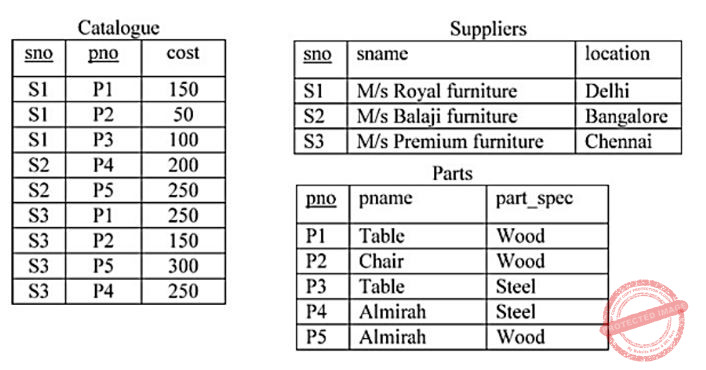The primary key of each table is indicated by underlying the constituent fields.

```SELECT s.sno, s.sname
FROM Suppliers s, Catalogue c
WHERE s.sno = c.sno AND
Cost > (SELECT AVG (cost)
FROM Catalogue
WHERE pno = ‘P4’
GROUP BY pno);```

The number of rows returned by the above SQL query is

 A 0 B 5 C 4 D 2
Database-Management-System       SQL       GATE 2020       Video-Explanation
Question 39 Explanation:
The inner query “select avg(cost) from catalogue where pno='P4' group by pno;” returns:
AVG(COST)
------------
225
The outer query “select s.sno, s.sname from suppliers s, catalogue c where s.sno=c.sno” returns:
SNO SNAME
----------------------------------------
S1 M/s Royal furniture
S1 M/s Royal furniture
S1 M/s Royal furniture
S2 M/s Balaji furniture
S2 M/s Balaji furniture
So, the final result of the query is:
SN SNAME
----------------------------------------
S2 M/s Balaji furniture
Therefore, 4 rows will be returned by the query.
 Question 40

The following relations are used to store data about students, courses, enrollment of students in courses and teachers of courses. Attributes for primary key in each relation are marked by ‘*’.

``` Students (rollno*, sname, saddr)
courses (cno*, cname)
teach(tno*,tname,cao*) ```

(cno is course number, cname is course name, tno is teacher number, tname is teacher name, sname is student name, etc.)

Write a SQL query for retrieving roll number and name of students who got A grade in at least one course taught by teacher named Ramesh for the above relational database.

 A Theory Explanation.
Database-Management-System       SQL       GATE 1993
There are 40 questions to complete.

Register Now